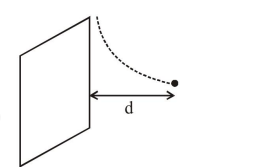# Solve this following

Question:

A particle of charge $\mathrm{q}$ and mass $\mathrm{m}$ is moving with a velocity $-v \hat{i}(v \neq 0)$ towards a large screen placed in the Y-Z plane at a distance $d$. If there is a magnetic field $\overrightarrow{\mathrm{B}}=\mathrm{B}_{0} \hat{\mathrm{k}}$, the minimum value of $v$ for which the particle will not hit the screen is:

1. $\frac{\mathrm{qdB}_{0}}{2 \mathrm{~m}}$

2. $\frac{q d B_{0}}{m}$

3. $\frac{2 \mathrm{qdB}_{0}}{\mathrm{~m}}$

4. $\frac{\mathrm{qdB}_{0}}{3 \mathrm{~m}}$

Correct Option: , 2

Solution:In uniform magnetic field particle moves in a circular path, if the radius of the circular path is 'd', particle will not hit the screen.

$\mathrm{d}=\frac{\mathrm{mv}}{\mathrm{qB}_{0}}$

$v=\frac{q B_{0} d}{m}$

$\therefore$ correct option is (2)